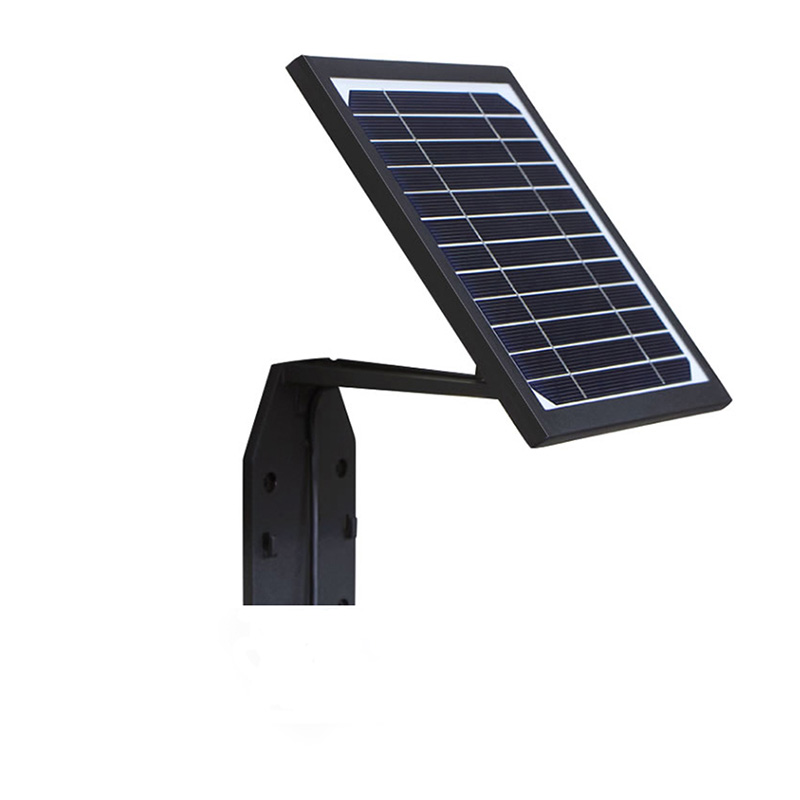Industry News

2021-05-13

# How many kilowatt-hours of electricity can the RV Solar Panel board generate in a day?

"How much electricity can the Solar Panel generate in a day" is really a question that many car riders are very concerned about. Before that, let's first understand what are the main factors that affect the power

generation efficiency of Solar Panel?

The first is the angle of illumination. We know that the sun rises in the east and sets in the west, and the solar panel has different power generation efficiency at different illumination angles. Its power

generation efficiency is the highest under the condition of vertical illumination;

The second is the light intensity, which can be more intuitively felt through the experimental data in the figure below. In the figure below, different colors represent different light intensities, and the output

power of the same Solar Panel under different light intensities is different. For example, the maximum output power of the Solar Panel is about 101w under the light intensity of 1200 lux, while the maximum

output power under the light intensity of 200 lux is only about 17w, which is more than 5 times the difference. It can also be seen from the figure that the light The greater the intensity, the greater the working

current of the solar panel.## Knowing the main factors that affect the power generation efficiency of the Solar Panel, how much power can the Solar Panel generate in a day?

If you want to ask how much electricity the Solar Panel can generate in a day? Under the influence of so many factors, it is actually difficult to give an absolutely definite number. But we can make a theoretical calculation:

a 100-watt Solar Panel can theoretically emit 100W*6H=600WH= under standard lighting conditions (light intensity of 1000W per square meter) and an average sunshine duration of 6 hours. 0.6KWH=0.6 kWh of electricity.

After all, there is a gap between theory and reality. This figure must be discounted in real scenarios. If we calculate various losses (line loss, light angle, light intensity, etc.), we calculate with a conversion rate of 70% A 100-watt

Solar Panel can generate approximately 0.6*70%=0.42 kWh per day.